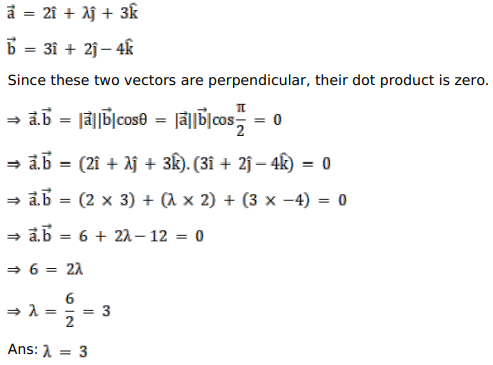# Solve this following

Question:

Find the value of $\lambda$ for which the vectors $(2 \hat{i}+\lambda \hat{j}+3 \hat{k})$ and $(3 \hat{i}+2 \hat{j}-4 \hat{k})$ are perpendicular to each other.

Solution: